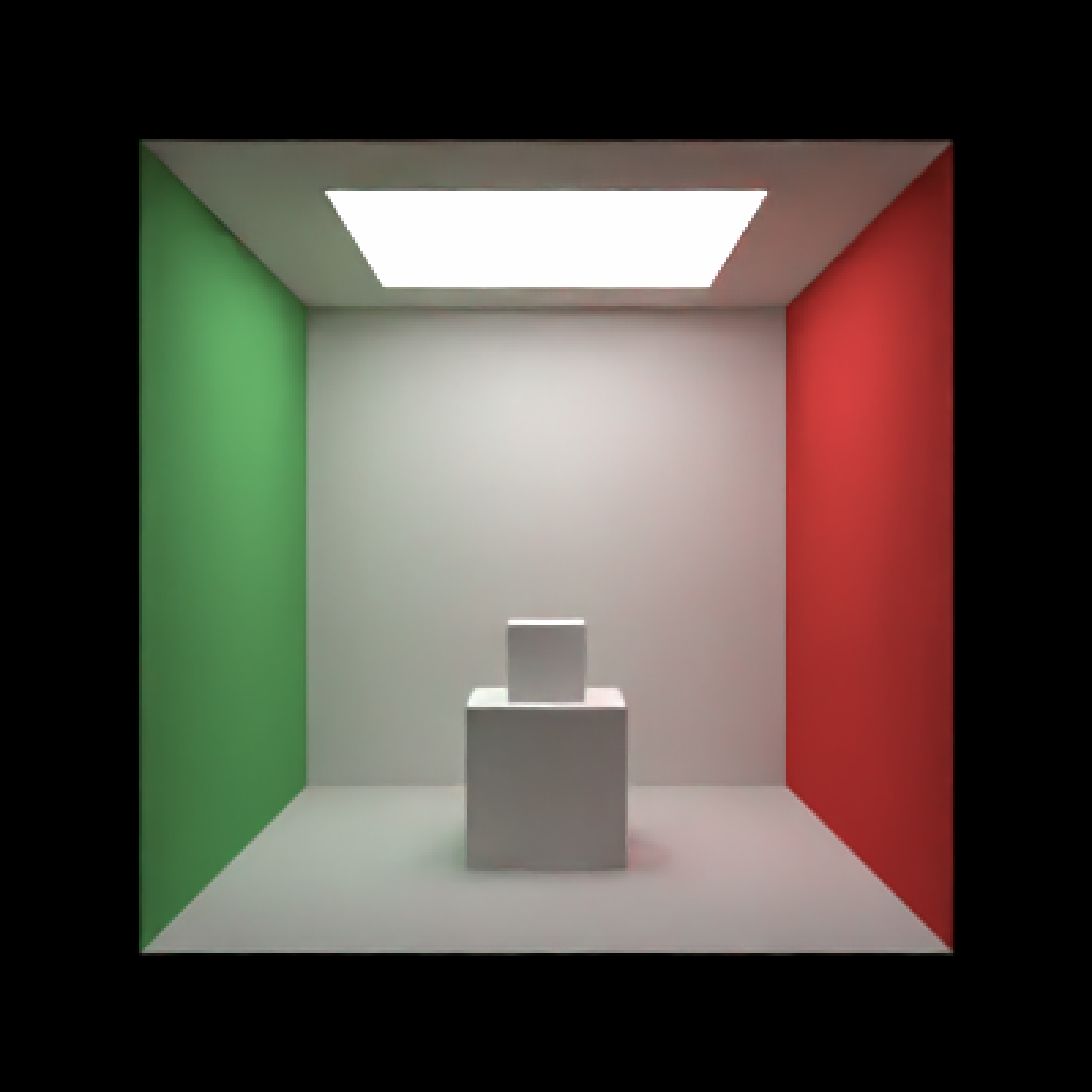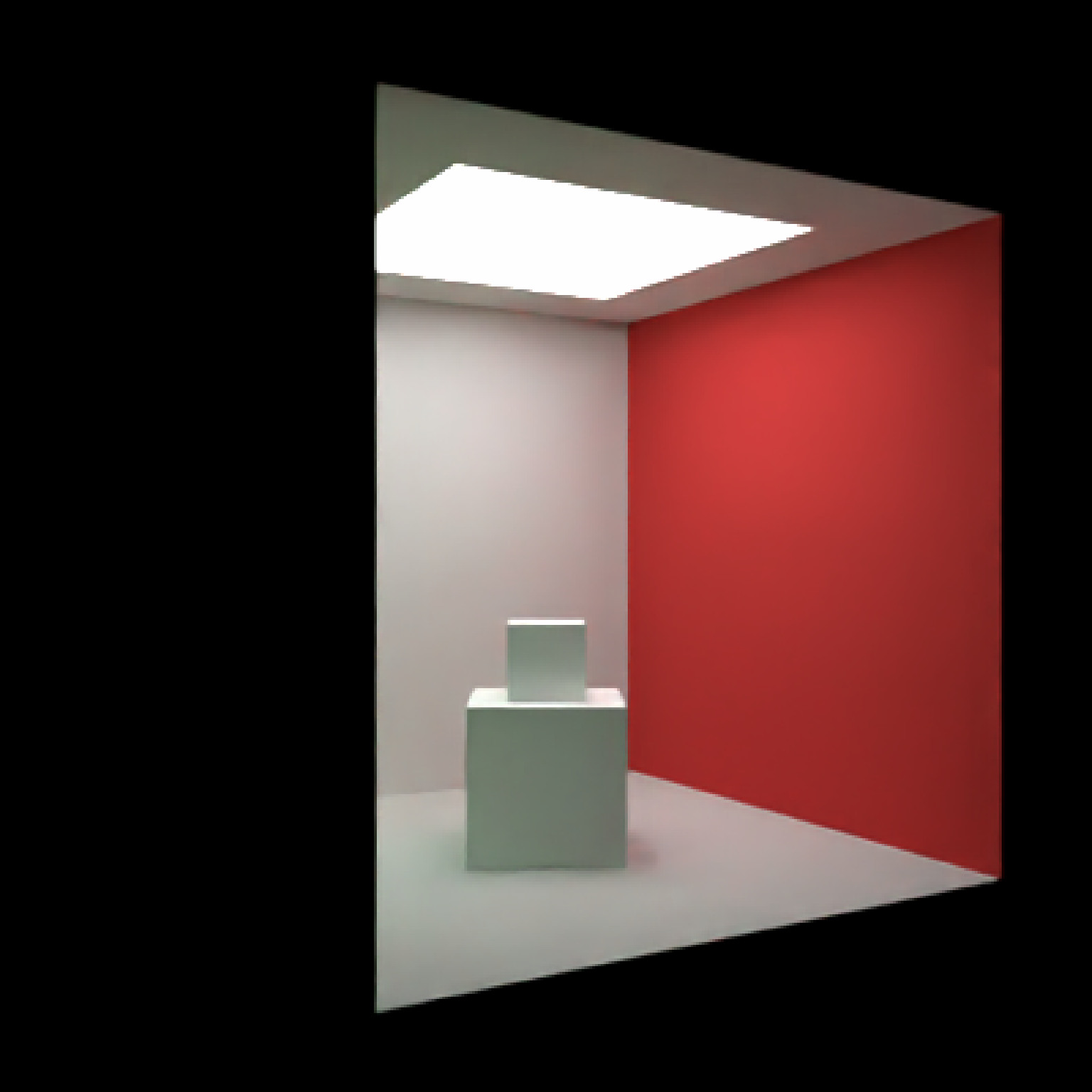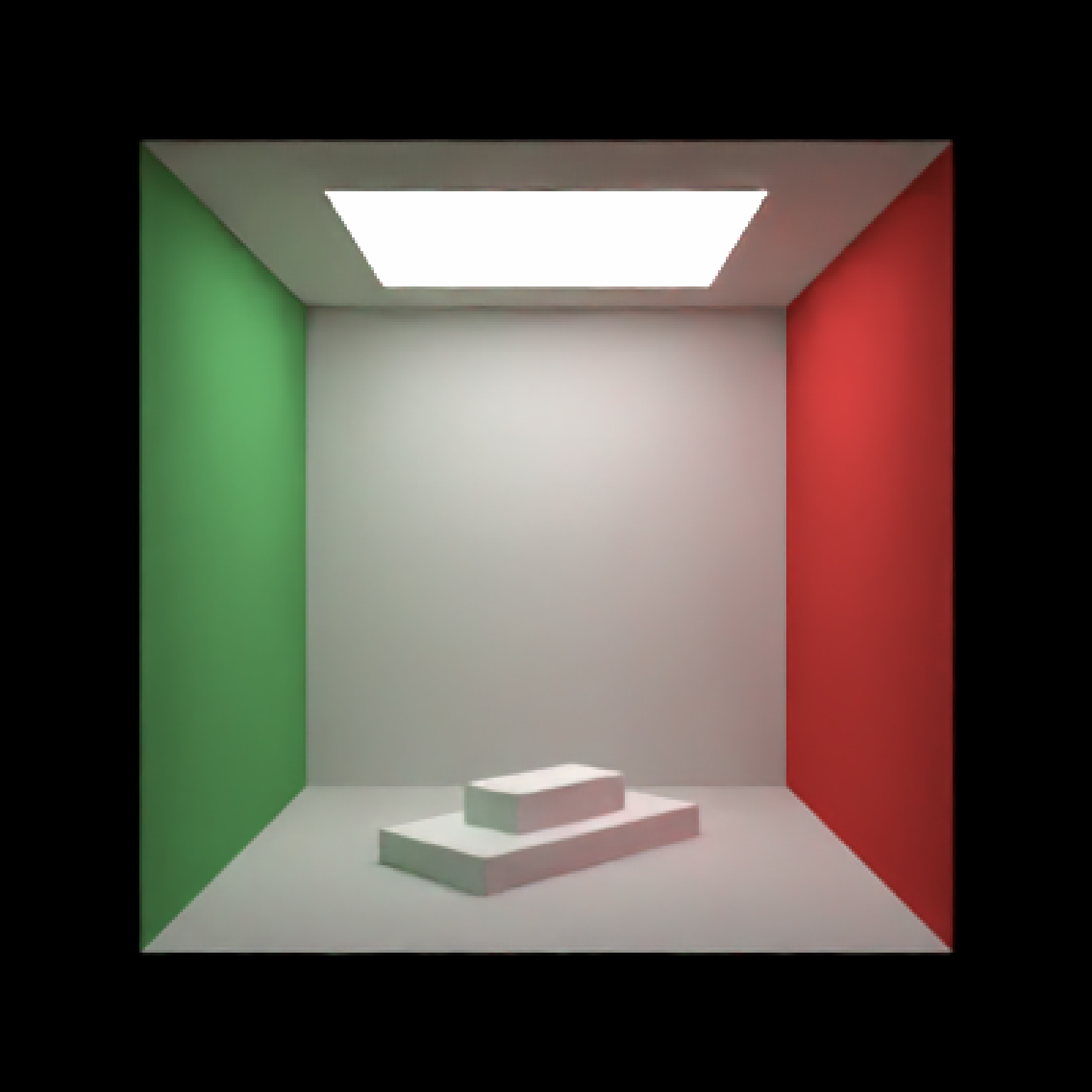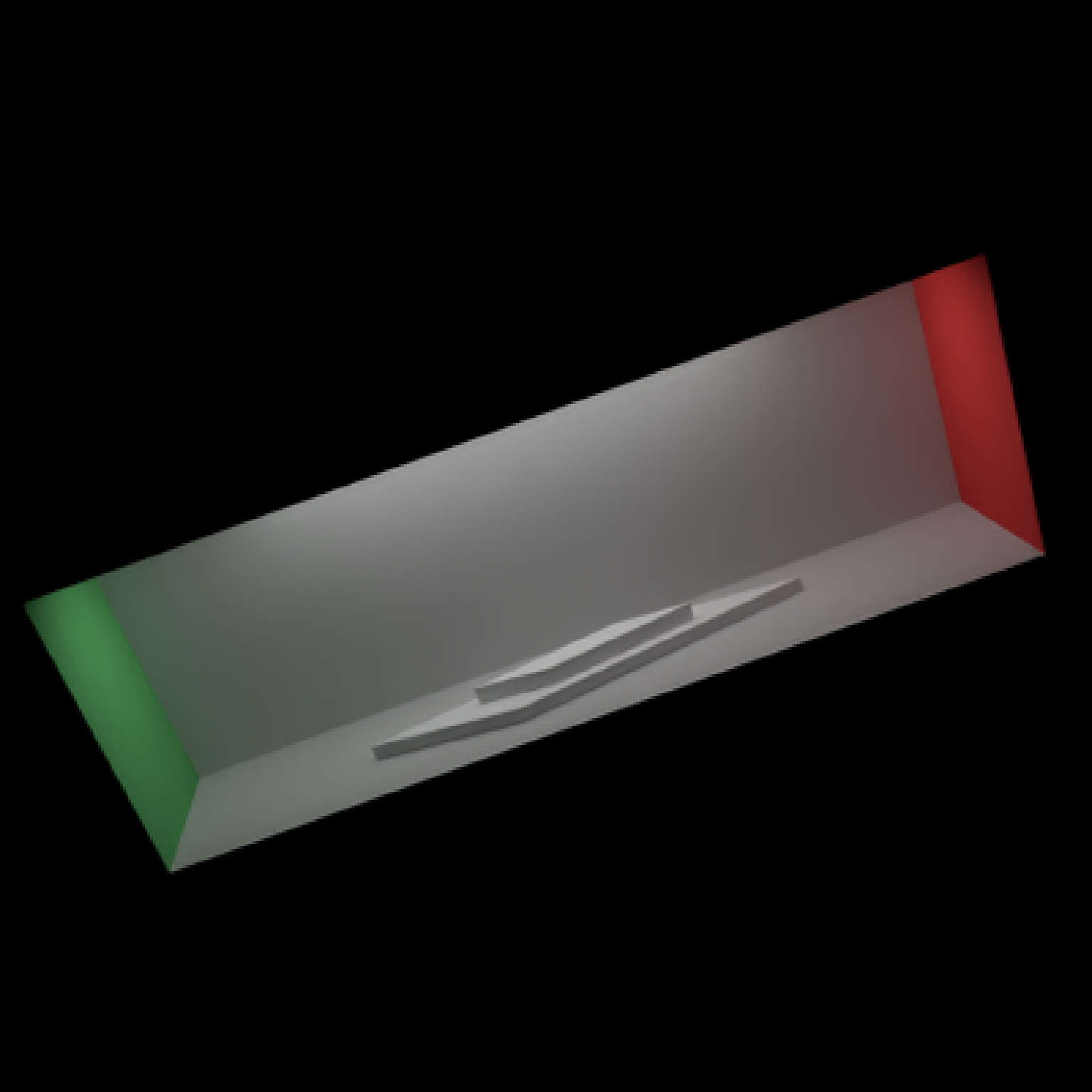Group and transform objects together.

group_objects(
scene,
pivot_point = c(0, 0, 0),
translate = c(0, 0, 0),
angle = c(0, 0, 0),
order_rotation = c(1, 2, 3),
scale = c(1, 1, 1),
axis_rotation = NA
)

## Arguments

scene

Tibble of pre-existing object locations and properties to group together.

pivot_point

Default c(0,0,0). The point about which to pivot, scale, and move the group.

translate

Default c(0,0,0). Vector indicating where to offset the group.

angle

Default c(0,0,0). Angle of rotation around the x, y, and z axes, applied in the order specified in order_rotation.

order_rotation

Default c(1,2,3). The order to apply the rotations, referring to "x", "y", and "z".

scale

Default c(1,1,1). Scaling factor for x, y, and z directions for all objects in group.

axis_rotation

Default NA. Provide an axis of rotation and a single angle (via angle) of rotation around that axis.

## Value

Tibble of grouped object locations and properties.

## Examples

#Generate the ground and add some objects
if(run_documentation()) {
scene = generate_cornell() %>%
render_scene(scene,lookfrom=c(278,278,-800),lookat = c(278,278,0), aperture=0,
samples=128, fov=50, parallel=TRUE, clamp_value=5)
}if(run_documentation()) {

#Group the entire room and rotate around its center, but keep the cubes in the same place.
scene2 = group_objects(generate_cornell(),
pivot_point=c(555/2,555/2,555/2),
angle=c(0,30,0)) %>%

render_scene(scene2,lookfrom=c(278,278,-800),lookat = c(278,278,0), aperture=0,
samples=128, fov=50, parallel=TRUE, clamp_value=5)
}if(run_documentation()) {
#Now group the cubes instead of the Cornell box, and rotate/translate them together
twocubes = cube(x=555/2,y=555/8,z=555/2,width=555/4) %>%
add_object(cube(x=555/2, y=555/4 + 555/16, z=555/2, width=555/8))
scene3 = generate_cornell() %>%
add_object(group_objects(twocubes, translate = c(0,50,0),angle = c(0,45,0),
pivot_point = c(555/2,0,555/2)))

render_scene(scene3,lookfrom=c(278,278,-800),lookat = c(278,278,0), aperture=0,
samples=128, fov=50, parallel=TRUE, clamp_value=5)
}if(run_documentation()) {
#Flatten and stretch the cubes together on two axes
scene4 = generate_cornell() %>%
angle = c(0,45,0), scale = c(2,0.5,1),
pivot_point = c(555/2,0,555/2)))

render_scene(scene4,lookfrom=c(278,278,-800),lookat = c(278,278,0), aperture=0,
samples=128, fov=50, parallel=TRUE, clamp_value=5)
}if(run_documentation()) {
#Add another layer of grouping, including the Cornell box
scene4 %>%
group_objects(pivot_point = c(555/2,555/2,555/2),scale=c(1.5,0.5,0.3), angle=c(-20,0,20)) %>%
render_scene(lookfrom=c(278,278,-800),lookat = c(278,278,0), aperture=0,
samples=509, fov=50, parallel=TRUE, clamp_value=5)
}